# Sum and Difference Identities Sum and Difference Identities

• Slides: 11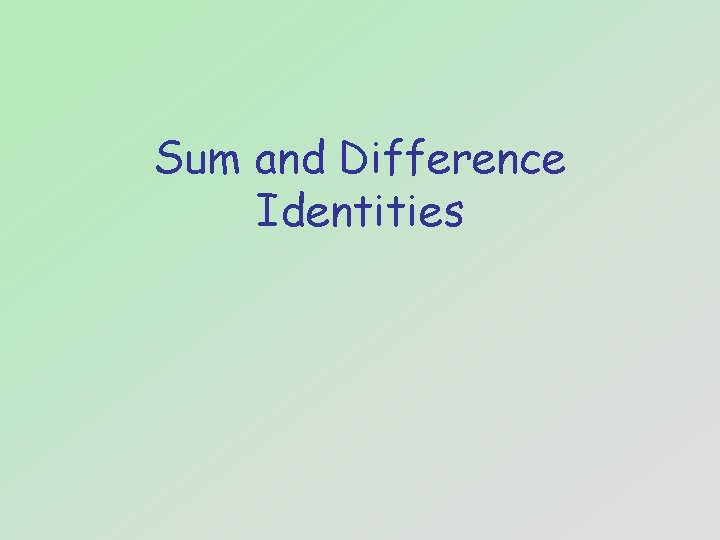Sum and Difference Identities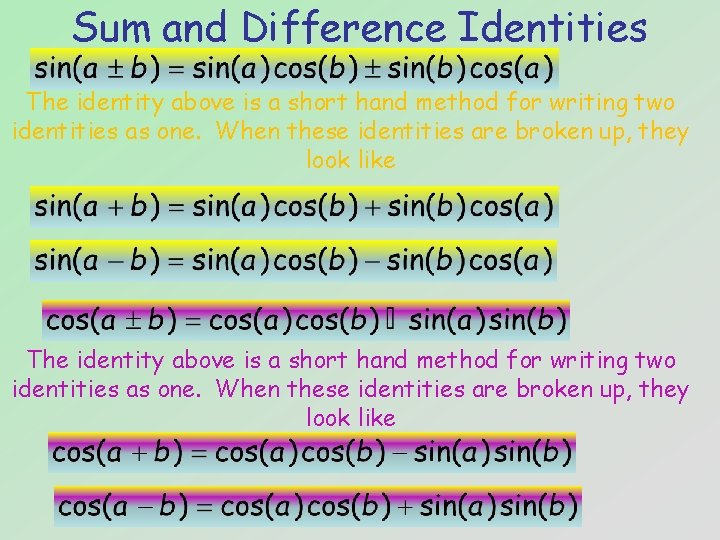Sum and Difference Identities The identity above is a short hand method for writing two identities as one. When these identities are broken up, they look like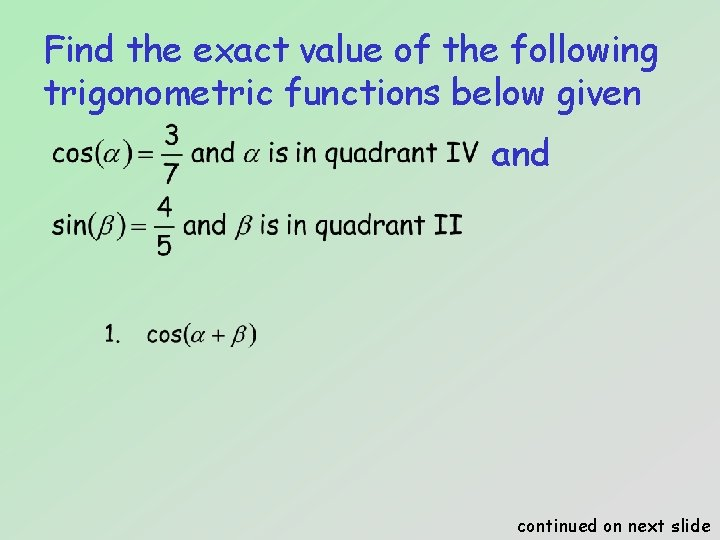Find the exact value of the following trigonometric functions below given and continued on next slide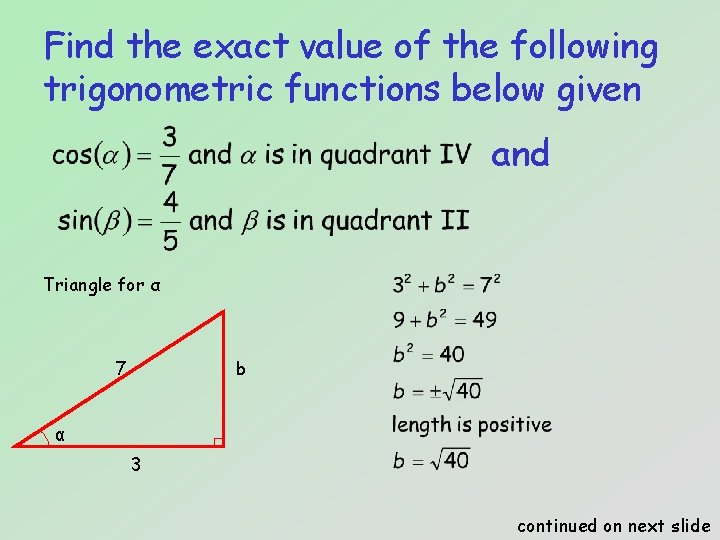Find the exact value of the following trigonometric functions below given and Triangle for α 7 b α 3 continued on next slide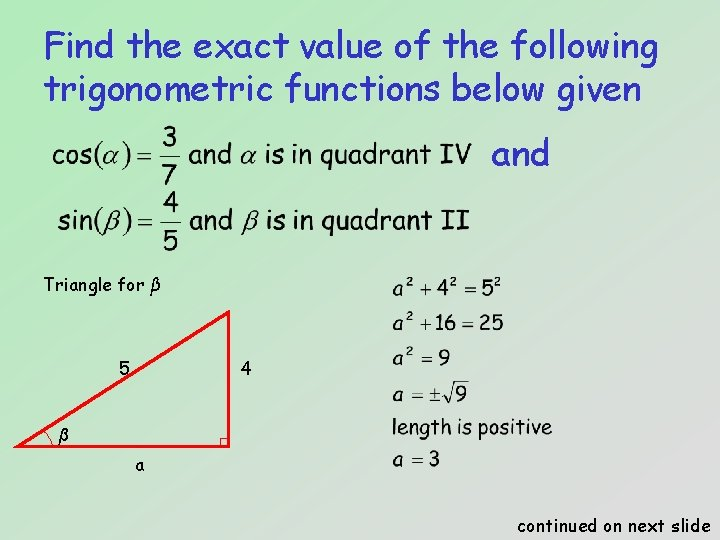Find the exact value of the following trigonometric functions below given and Triangle for β 5 4 β a continued on next slide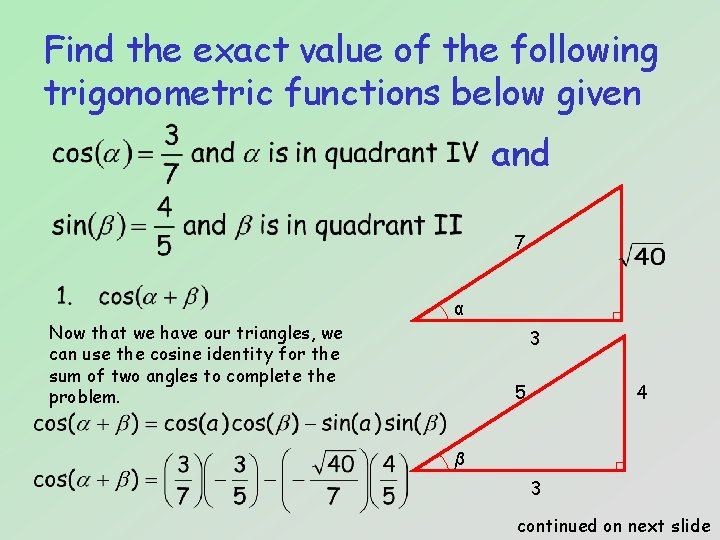Find the exact value of the following trigonometric functions below given and 7 Now that we have our triangles, we can use the cosine identity for the sum of two angles to complete the problem. α 3 5 4 β 3 continued on next slide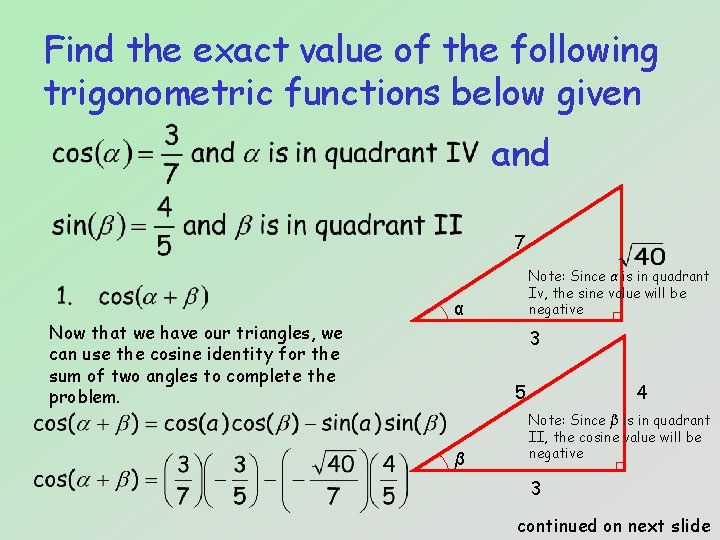Find the exact value of the following trigonometric functions below given and 7 Now that we have our triangles, we can use the cosine identity for the sum of two angles to complete the problem. Note: Since α is in quadrant Iv, the sine value will be negative α 3 5 β 4 Note: Since β is in quadrant II, the cosine value will be negative 3 continued on next slideFind the exact value of the following trigonometric functions below given and 7 α 3 5 4 β 3 continued on next slideFind the exact value of the following trigonometric functions below given and continued on next slide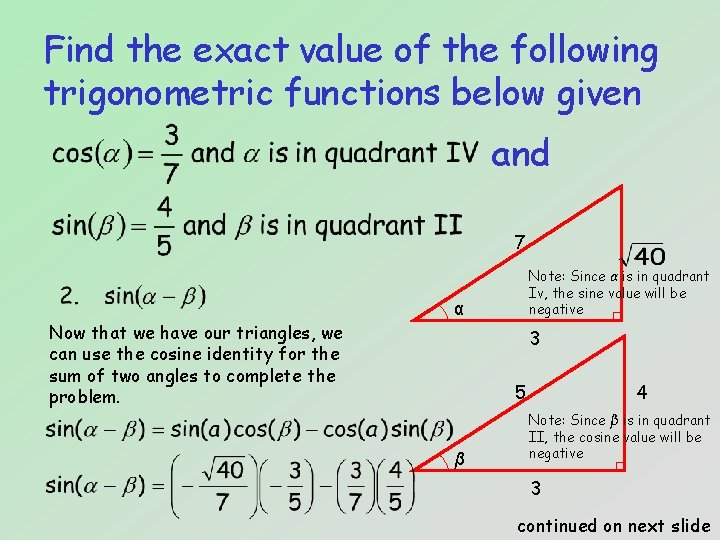Find the exact value of the following trigonometric functions below given and 7 Now that we have our triangles, we can use the cosine identity for the sum of two angles to complete the problem. Note: Since α is in quadrant Iv, the sine value will be negative α 3 5 β 4 Note: Since β is in quadrant II, the cosine value will be negative 3 continued on next slideFind the exact value of the following trigonometric functions below given and 7 α 3 5 4 β 3